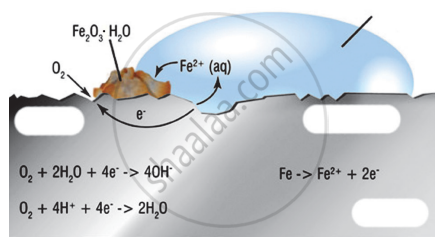# Observe the following figure and write the answer of the question. 1. Which process is shown in the figure? 2. Explain the chemical reaction shown in the figure. - Science and Technology 1

Observe the following figure and write the answer of the question.1. Which process is shown in the figure?
2. Explain the chemical reaction shown in the figure.
3. Write the reactions on anode and cathode.

#### Solution

1. The process shown in the figure is rusting (corrosion) of iron.
2. The process involves electrochemical reaction. Different regions on the surface of iron become anode and cathode. In the anode region, Fe is oxidized to Fe2+. The electron released in the anode region flow through the metal surface to the cathode region where they reduce oxygen.
In the cathode region, O2 is reduced to form water. When Fe2+ ions migrate from the anode region, they react with water (or OH ions) and further get oxidized to Fe3+ ions. Fe3+ ions form an insoluble hydrated oxide (Fe2O3.H2O), which is deposited as reddish-brown layer on the surface. It is called rust.
$\ce{2F^3+_{(aq)} + 4H2O_{(l)} -> \underset{\text{Rust}}{Fe2O3.H2O_{(s)}} + 6H+_{(aq)}}$
3. Anode region: $\ce{Fe_{(s)} -> Fe^2+_{(aq)} + 2e^-}$
Cathode region: $\ce{O_{2(g)} + 4H^+_{(aq)} + 4e^- -> 2H2O_{(l)}}$
Concept: Corrosion of Metals and Its Prevention
Is there an error in this question or solution?

#### APPEARS IN

SCERT Maharashtra Question Bank 10th Standard SSC Science and Technology 1 Maharashtra State Board 2021
Chapter 3 Chemical reactions and equations
Answer the following questions | Q 2